Bombieri-Iwaniec method

Invented in [a1] and [a2], where it was used to bound the Riemann zeta-functionalong the mid-line of its critical strip, this is currently (2000) the strongest method around for establishing upper bounds on several wide classes of Weyl sums that have many applications within analytic number theory (cf. also Weyl sum). General application to the first such class, exponential sums within the scope of Van der Corput's exponent pairs theory (see [a3]), was done by M.N. Huxley and N. Watt in [a10]. Improvements to one aspect of the method (the first spacing problem below) were found by Watt [a16] and Huxley and G. Kolesnik [a8], while in [a11] H. Iwaniec and C.J. Mozzochi made an important adaptation, forming a second branch of the method that yielded new results for the circle and divisor problems. In a series of papers beginning with [a4] Huxley has generalized and refined this adaptation, applying it to bound lattice point discrepancy (the difference between an area and the number of integer lattice points within the area; cf. also Lattice of points; Geometry of numbers). The original insight of E. Bombieri and Iwaniec into the second spacing problem (see below), which is of crucial importance for both branches of the method, has been significantly augmented by the theory of resonance curves invented and refined by Huxley in [a5] and [a7].

See [a3] for a brief introduction to the method; [a6] is the most recent book on the method, and covers almost all of its aspects, including applications to, e.g.: exponential sums with a Dirichlet character factor; the mean-square of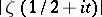over a short interval; and counting integer lattice points near a curve. It also covers Jutila's independent (but related) method of [a12] and [a13], used for the estimation of exponential sums with a Fourier coefficient of a cusp form, or a divisor function, as a factor.

The original branch of the Bombieri–Iwaniec method deals with a sum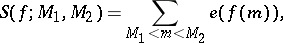where,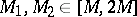, and the derivatives (from first to fourth, say) ofhave absolute values ranging within a uniformly bounded factor of a single parameter(sufficiently large) with. The method, which only applies directly when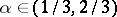, has five main steps:

i) Division of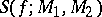into consecutive shorter sums within each of which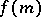is approximated, via Taylor series, by a cubic polynomial with rational quadratic coefficient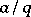.

ii) Use of Poisson summation (cf. also Poisson summation method), evaluation of Gauss sums (cf. also Gauss sum) and the stationary phase method (cf. also Stationary phase, method of the) to transform the short sum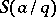belonging tointo an even shorter one: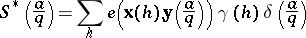with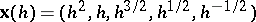and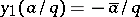, where.

iii) Estimation of the sum overof, in terms of theth power moment, using the Hölder inequality and the double large sieve introduced in [a1] (cf. also Large sieve). This leaves two steps to provide data for the sieve.

iv) The first spacing problem: Count pairs of "neighbours" amongst vectors of the form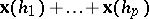.

v) Second spacing problem: Count pairs of "neighbours"and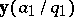(coincidences). Steps iv) and v) remain open problems (as of 2000), as does the question of more radical improvement of the whole. E. Fouvry and Iwaniec, using only Van der Corput's method with the double large sieve from Step iii), found useful new bounds for their monomial exponential sums (see [a6]).

The method's second branch treatswhere the summation is over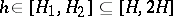and,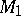and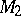are as above. Sincehas been replaced by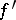, so must the cubic approximation of Step i) be replaced by its derivative. Steps ii)–iv) also change, but the second spacing problem does not. These sums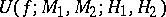can arise when a truncated form of the Fourier series for the sawtooth functionis used in the estimation of the lattice point discrepancy of an area of size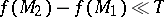bounded by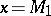,,and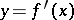in the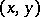-plane. Ifgets too large, then one can switch to summing the discrepancies for columns of widthto summing those for rows of depth, the number of which required will be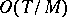. Poisson summation makes a similar switch possible in estimatingalone. In both contexts the critical cases are those around(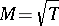), so the, being values of, will satisfy; something like the situation in applications of the Hardy–Littlewood circle method.

Jutila's method [a13] utilizes a "twisted" Wilton summation formula, corresponding to a cusp form of even weightfor the full modular group: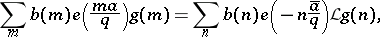where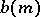is theth Fourier coefficient of the cusp form,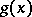is a smooth function supported in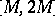, and the linear integral transformhas integrand containing the Bessel-function of order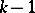as a factor (cf. also Bessel functions). Step ii) above is essentially the extension of Jutila's formula to the case where the cusp form is replaced by the theta-series of weight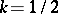with Fourier coefficients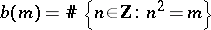. M. Jutila has an analogous "twisted" Voronoi summation formula for the more delicate case whereis the divisor function (cf. also Number of divisors), for which the corresponding modular form is non-holomorphic; this enabled a more elementary proof of a famous theorem of Iwaniec (see [a14]).

The original treatment by Bombieri and Iwaniec of the second spacing problem rested on the observation that proximity of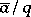and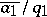(modulo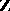) implies that the row vectorsand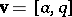will be linked by a relation, with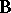being an integer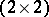-matrix of determinanthaving a "small" lower left entry. Huxley's theory of resonance curves showed that the coincident pairs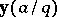,for a given choice ofcorrespond to integer points lying near a certain plane resonance curve, this curve being determined (up to a minor transformation) by the choice of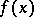and. This led, in [a5], to better bounds in the second spacing problem, to improved exponential sum estimates, and (for example) to the result that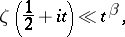when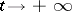, with fixed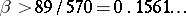(an unpublished improvement of this and of resonance curve theory is given in [a7]). For comparison, Bombieri and Iwaniec's original paper [a1] had, while even a complete resolution of both the first and second spacing problems (alone) could not getbelow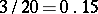.

Integer points near a suitable curve may be counted using exponential sum estimates, so resonance curves invite one to apply the Bombieri–Iwaniec method iteratively. P. Sargos managed to do this in [a15], using his own simpler theory of (quite different) resonance curves. He obtained results of greatest interest fornear. A third construction of resonance curves (different again) has been given by Huxley and Kolesnik [a9], and is of interest fornear. They were able to apply this iteratively, but got better results (in most cases) with a single step of an elementary method once employed by H. Swinnerton-Dyer to count lattice points exactly on a curve.

How to Cite This Entry:
Bombieri-Iwaniec method. Encyclopedia of Mathematics. URL: http://encyclopediaofmath.org/index.php?title=Bombieri-Iwaniec_method&oldid=17481
This article was adapted from an original article by N. Watt (originator), which appeared in Encyclopedia of Mathematics - ISBN 1402006098. See original article